Next: Square Potential Well Up: One-Dimensional Potentials Previous: Cold Emission

# Alpha Decay

Many types of heavy atomic nucleus spontaneously decay to produce daughter nucleii via the emission of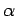-particles (i.e., helium nucleii) of some characteristic energy. This process is know as-decay. Let us investigate the-decay of a particular type of atomic nucleus of radius, charge-number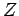, and mass-number. Such a nucleus thus decays to produce a daughter nucleus of charge-numberand mass-number, and an-particle of charge-number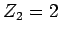and mass-number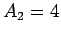. Let the characteristic energy of the-particle be. Incidentally, nuclear radii are found to satisfy the empirical formula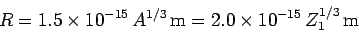(353)

for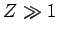.

In 1928, George Gamov proposed a very successful theory of-decay, according to which the-particle moves freely inside the nucleus, and is emitted after tunneling through the potential barrier between itself and the daughter nucleus. In other words, the-particle, whose energy is, is trapped in a potential well of radiusby the potential barrier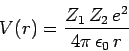(354)

for.

Making use of the WKB approximation (and neglecting the fact thatis a radial, rather than a Cartesian, coordinate), the probability of the-particle tunneling through the barrier is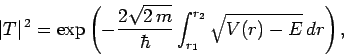(355)

where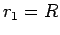and. Here,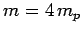is the-particle mass. The above expression reduces to(356)

where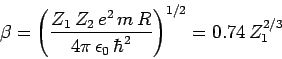(357)

is a dimensionless constant, and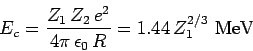(358)

is the characteristic energy the-particle would need in order to escape from the nucleus without tunneling. Of course,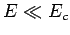. It is easily demonstrated that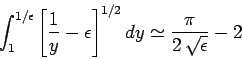(359)

when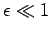. Hence.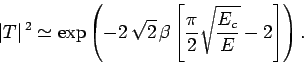(360)

Now, the-particle moves inside the nucleus with the characteristic velocity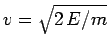. It follows that the particle bounces backward and forward within the nucleus at the frequency, giving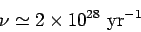(361)

for a 1 MeV-particle trapped inside a typical heavy nucleus of radiusm. Thus, the-particle effectively attempts to tunnel through the potential barrier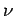times a second. If each of these attempts has a probability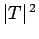of succeeding, then the probability of decay per unit time is. Hence, if there are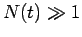undecayed nuclii at timethen there are only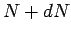at time, where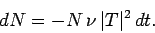(362)

This expression can be integrated to give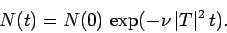(363)

Now, the half-life,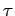, is defined as the time which must elapse in order for half of the nuclii originally present to decay. It follows from the above formula that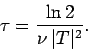(364)

Note that the half-life is independent of.

Finally, making use of the above results, we obtain(365)

where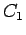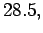(366)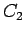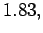(367)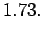(368)The half-life,, the daughter charge-number,, and the-particle energy,, for atomic nucleii which undergo-decay are indeed found to satisfy a relationship of the form (365). The best fit to the data (see Fig. 15) is obtained using(369)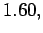(370)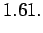(371)

Note that these values are remarkably similar to those calculated above.Next: Square Potential Well Up: One-Dimensional Potentials Previous: Cold Emission
Richard Fitzpatrick 2010-07-20